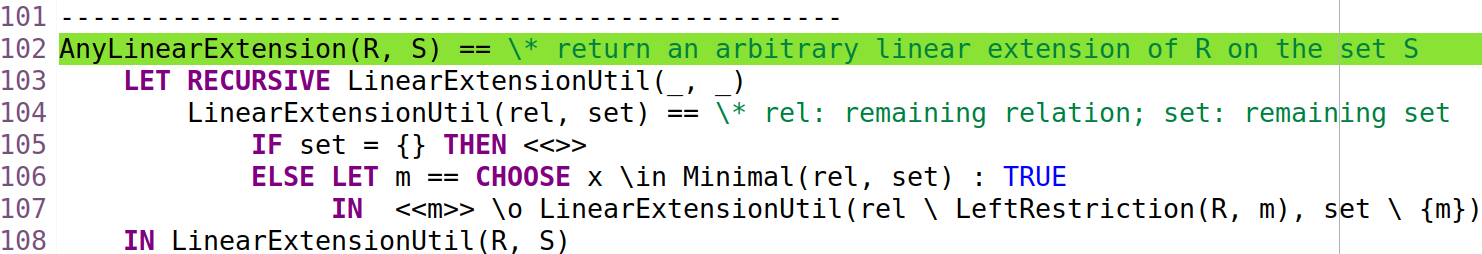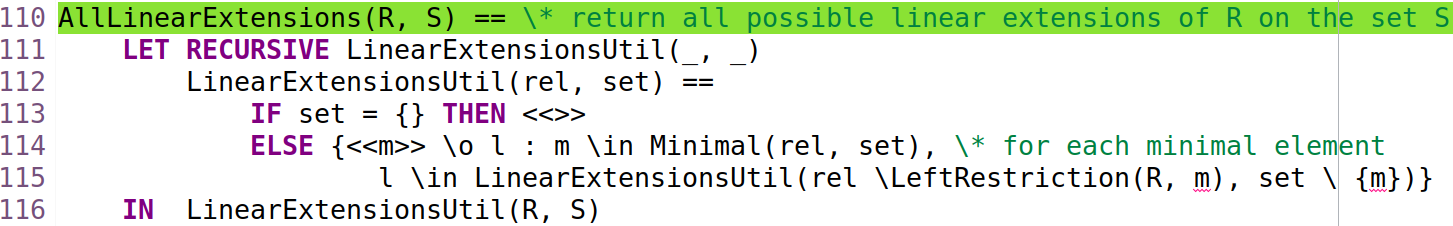# [tlaplus] How to generate all possible linear extensions (i.e., topological sortings) of a partial order?

Hi All,

I am writing a TLA+ specification of a consistency model which is defined based on relations. I need to generate all possible linear extensions (i.e., topological sortings) of a partial order.

The following TLA+ code can be found at https://github.com/hengxin/tla-causal-consistency/blob/main/RelationUtils.tla.

I am able to generate an arbitrary linear extension of a partial order as follows (I use this algorithm: https://en.wikipedia.org/wiki/Topological_sorting#Kahn's_algorithm):where Minimal(R, S) returns the set of minimal elements of S given relation R,
and LeftRestriction(R, m) returns the set of pairs whose first element is m.

However, I failed to generate all possible linear extensions of a partial order:TLC reports an error: Unknown operator 'm'.

So how to generate all possible linear extensions of a partial order?

Best regards,
Hengfeng Wei (hengxin)

--
You received this message because you are subscribed to the Google Groups "tlaplus" group.
To unsubscribe from this group and stop receiving emails from it, send an email to tlaplus+unsubscribe@xxxxxxxxxxxxxxxx.